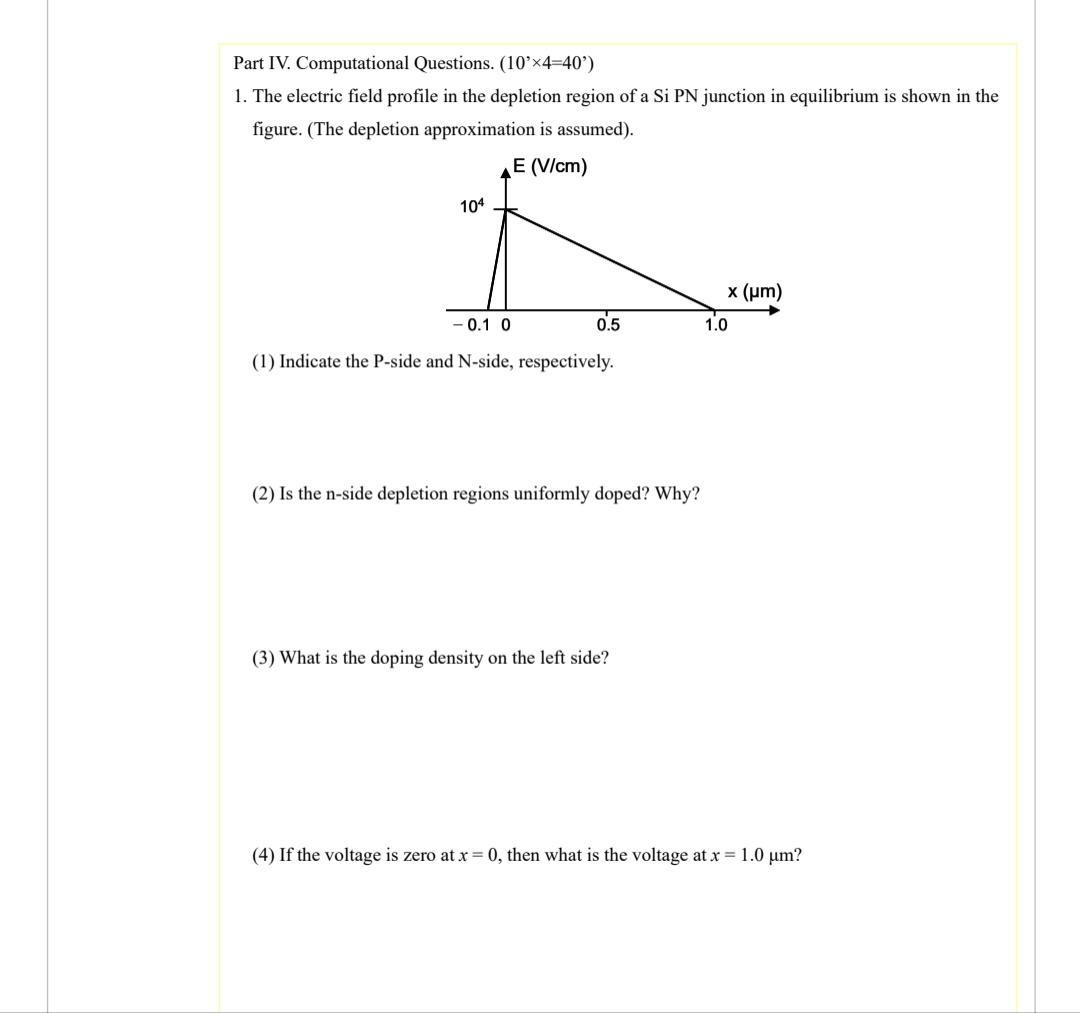Home / Expert Answers / Electrical Engineering / part-iv-computational-questions-left-10-prime-times-4-40-prime-right-1-the-elect-pa959

# (Solved): Part IV. Computational Questions. $$\left(10^{\prime} \times 4=40^{\prime}\right)$$ 1. The elect ...Part IV. Computational Questions. $$\left(10^{\prime} \times 4=40^{\prime}\right)$$ 1. The electric field profile in the depletion region of a Si PN junction in equilibrium is shown in the figure. (The depletion approximation is assumed). (1) Indicate the P-side and N-side, respectively. (2) Is the n-side depletion regions uniformly doped? Why? (3) What is the doping density on the left side? (4) If the voltage is zero at $$x=0$$, then what is the voltage at $$x=1.0 \mu \mathrm{m}$$ ?

We have an Answer from Expert